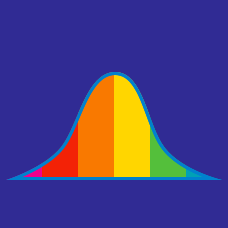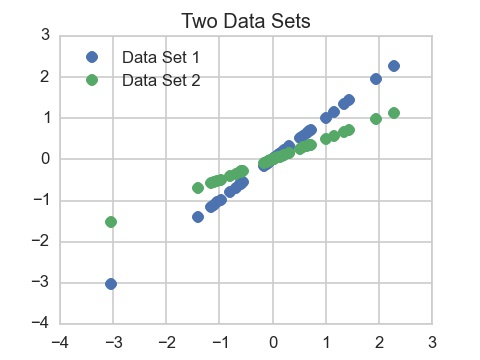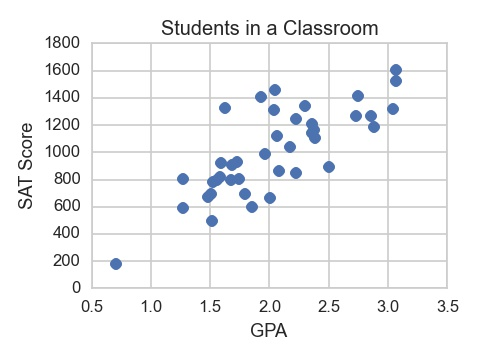Probability

# Correlation

What is the maximum value that the correlation of two random variables can take on?Consider the two data sets represented by the data in the graph. Suppose that for each data set, the points lie exactly on a line. Which one has a higher correlation?The scatter plot above is drawn to represent a class of 40 students. Which of the following is closest to the correlation between GPA and SAT score?

Johnny is measuring and weighing apples. He finds that the correlation between the width measured in inches to the weight measured in pounds is 0.2. What is the correlation between the width in centimeters and the weight in pounds?

You may find it helpful to know that there are 2.54 centimeters in an inch.

You have 100 squares all of which are a different size. What is the correlation between the area and side length of these squares?

×